﻿ 含函数型自变量回归模型中的变量选择<sup>*</sup>Download PDF文章快速检索 高级检索

1. 北京航空航天大学 学生大数据中心, 北京 100083;
2. 中央财经大学 统计与数学学院, 北京 100081

Variable selection in regression models including functional data predictors
LIU Kesheng1, WANG Siyang21. Big Data Center of Student Affairs Department, Beihang University, Beijing 100083, China;
2. School of Statistics and Mathematics, Central University of Finance and Economics, Beijing 100081, China
Received: 2019-04-11; Accepted: 2019-04-26; Published online: 2019-06-19 11:19
Foundation item: National Natural Science Foundation of China (11501586, 71420107025); Program for Innovation Research in Central University of Finance and Economics
Corresponding author. WANG Siyang, E-mail: siyangw@163.com
Abstract: The variable selection and parameter estimation problem is researched in the framework of mixed-type regression model with both functional and multivariate predictors, which broadens the scope of functional data analysis and the application fields of variable selection methodology. First the functional predictors are projected into spaces spanned by functional principal component basis functions. Then variable selection and parameter estimation are implemented simultaneously for the multivariate predictors and derived projection predictors in the form of grouping, where the tuning parameter of the penalized term is adaptively selected and the loss function is based on absolute median loss function. As to the optimization procedure, by introducing slack variables, it is transformed into a linear programming problem with several constraint conditions, which simplifies the computation. The simulation results illustrate that the proposed method performs quite well in variable selection and parameter estimation in the mixed-type regression model.
Keywords: functional data     variable selection     parameter estimation     quantile     functional principal component

1 函数型和多元向量混合回归模型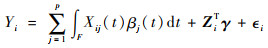(1)

2 模型变量选择和参数估计

2.1 函数型主成分及模型转化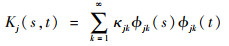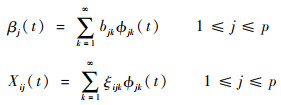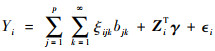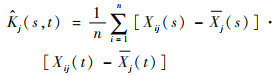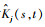进行类似谱分解可得到，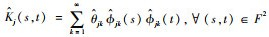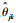为函数型谱分解中的特征根。注意，{Xij(t), 1≤in}张成的空间中任意一组基函数的维数不超过样本量n，所以若{}以降序排列，则有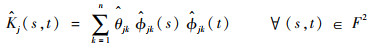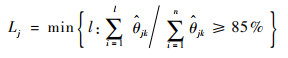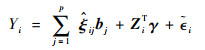(2)

2.2 参数估计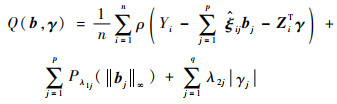(3)

2.3 调节参数选择及目标函数优化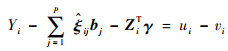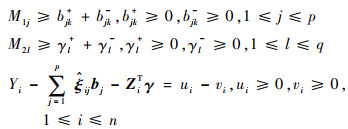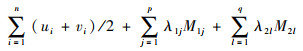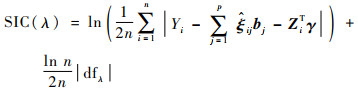3 数值模拟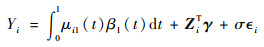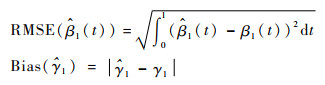(n, σ) 统计指标 TP FP RMSE Bias (100, 0.05) Mean 2 0.22 0.028 2 0.005 8 Sd 0 0.52 0.007 6 0.004 4 (100, 0.2) Mean 2 0.34 0.084 4 0.022 9 Sd 0 0.61 0.033 0 0.017 9 (300, 0.05) Mean 2 0.09 0.016 8 0.002 7 Sd 0 0.30 0.004 8 0.002 0 (300, 0.2) Mean 2 0.18 0.049 1 0.012 0 Sd 0 0.42 0.019 5 0.009 8

 (n, σ) 统计指标 TP FP RMSE Bias (100, 0.05) Mean 2 0.01 0.036 0 0.008 3 Sd 0 0.07 0.007 6 0.004 4 (100, 0.2) Mean 2 0.03 0.116 8 0.035 5 Sd 0 0.16 0.054 7 0.030 1 (300, 0.05) Mean 2 0 0.019 5 0.003 8 Sd 0 0 0.006 5 0.002 8 (300, 0.2) Mean 2 0.12 0.062 1 0.014 0 Sd 0 0.32 0.026 6 0.011 6

4 结论

1) 本文同时考虑了函数型自变量和多元向量自变量，拓展了函数型数据分析的应用领域，给出了一种新的数据混合回归模型。

2) 引入惩罚函数同时进行变量选择和参数估计，对函数型自变量引入了组变量选择方法，对经过函数型主成分分析投影后的函数型自变量具有选择效果。

3) 在变量选择过程中，将目标函数优化问题转化为线性优化问题，降低了参数估计的复杂性。

4) 在参数估计过程中考虑了异常值的影响，采用了稳健变量选择方法，扩大了适用性。

  FERRATY F. Recent advances in functional data analysis and related topics[M]. Berlin: Springer, 2011.  CHEN S T, XIAO L, STAICU A M. A smoothing-based goodness-of-fit test of covariance for functional data[J]. Biometrics, 2018, 75(2): 562-571.  CUEVAS A. A partial overview of the theory of statistics with functional data[J]. Journal of Statistical Planning and Inference, 2014, 147: 1-23. DOI:10.1016/j.jspi.2013.04.002  PARK J, AHN J. Clustering multivariate functional data with phase variation[J]. Biometrics, 2017, 73(1): 324-333. DOI:10.1111/biom.12546  KATO K. Estimation in functional linear quantile regression[J]. Annals of Statistics, 2012, 40(6): 3108-3136. DOI:10.1214/12-AOS1066  TIBSHIRANI R. Regression shrinkage and selection via the Lasso[J]. Journal of the Royal Statistical Society.Series B(Statistical Methodology), 1996, 58(1): 267-288.  HALL P, HOROWITZ J L. Methodology and convergence rates for functional linear regression[J]. Annals of Statistics, 2007, 35(1): 70-91.  HALL P, HOSSEINI-NASAB M. On properties of functional principal components analysis[J]. Journal of the Royal Statistical Society.Series B(Statistical Methodology), 2005, 68(1): 109-126.  LIN X, LU T, YAN F, et al. Mean residual life regression with functional principal component analysis on longitudinal data for dynamic prediction[J]. Biometrics, 2018, 74(4): 1482-1491. DOI:10.1111/biom.12876  HUANG L, ZHAO J, WANG H, et al. Robust shrinkage estimation and selection for functional multiple linear model through LAD loss[J]. Computational Statistics & Data Analysis, 2016, 103: 384-400.  QIAN J, SU L. Shrinkage estimation of common breaks in panel data models via adaptive group fused Lasso[J]. Journal of Econometrics, 2016, 191(1): 86-109. DOI:10.1016/j.jeconom.2015.09.004  VINCENT M, HANSEN N R. Sparse group lasso and high dimensional multinomial classification[J]. Computational Statistics & Data Analysis, 2014, 71: 771-786.  LIU X, LIN Y, WANG Z. Group variable selection for relative error regression[J]. Journal of Statistical Planning and Inference, 2016, 175: 40-50. DOI:10.1016/j.jspi.2016.02.006  WANG H J, LI D, HE X. Estimation of high conditional quantiles for heavy-tailed distributions[J]. Journal of the American Statistical Association, 2012, 107(500): 1453-1464. DOI:10.1080/01621459.2012.716382  BANG S, JHUN M. Simultaneous estimation and factor selection in quantile regression via adaptive sup-norm regularization[J]. Computational Statistics & Data Analysis, 2012, 56(4): 813-826.  WANG T, ZHU L. Consistent tuning parameter selection in high dimensional sparse linear regression[J]. Journal of Multivariate Analysis, 2011, 102(7): 1141-1151. DOI:10.1016/j.jmva.2011.03.007  HIROSE K, TATEISHI S, KONISHI S. Tuning parameter selection in sparse regression modeling[J]. Computational Statistics & Data Analysis, 2013, 59: 28-40.

#### 文章信息

LIU Kesheng, WANG Siyang

Variable selection in regression models including functional data predictors

Journal of Beijing University of Aeronautics and Astronsutics, 2019, 45(10): 1990-1994
http://dx.doi.org/10.13700/j.bh.1001-5965.2019.0157